## Light curves, radius and mass of the exoplanets of my different constelations

### HAT-P-25b - Aries

Radius: Rp = √ 1 - 0,9778 · 0.959 = 0,142888RJ · 100 = 14,2888% more big than the earth radius. Compared to academic google results and NASA files, the radius is 1,19RJ and the mass is 0.567MJ.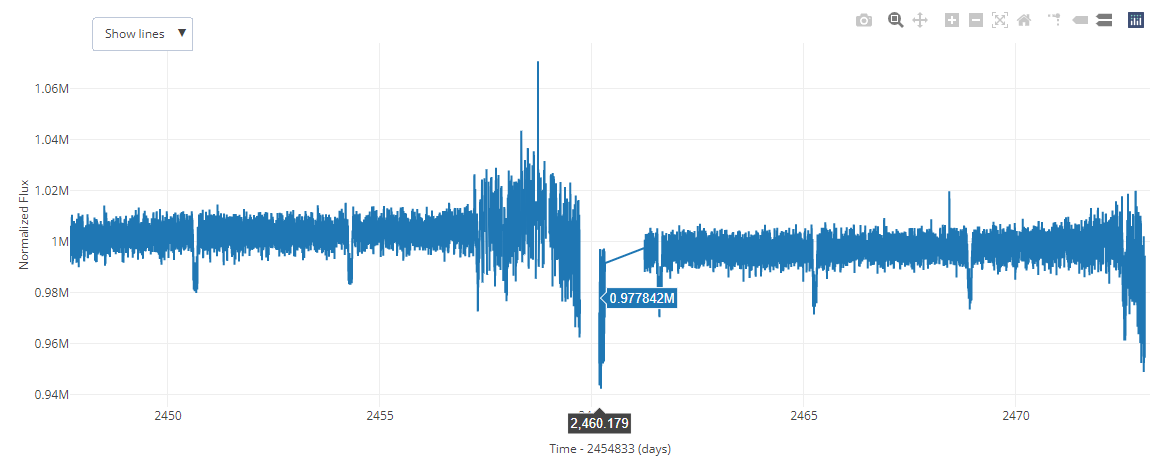Light Curve of HAT-P-25b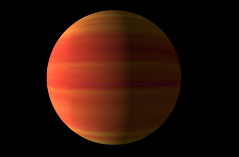HAT-P-25b virtual representation

### KELT-11b - Aquila

Radius: Rp = √ 1 - 0,9963 · 2.72 = 0,165451RJ · 100 = 16,5451% more big than the earth radius. Compared to academic google results and NASA files, the radius is 1.3RJ and the mass is 0.195MJ.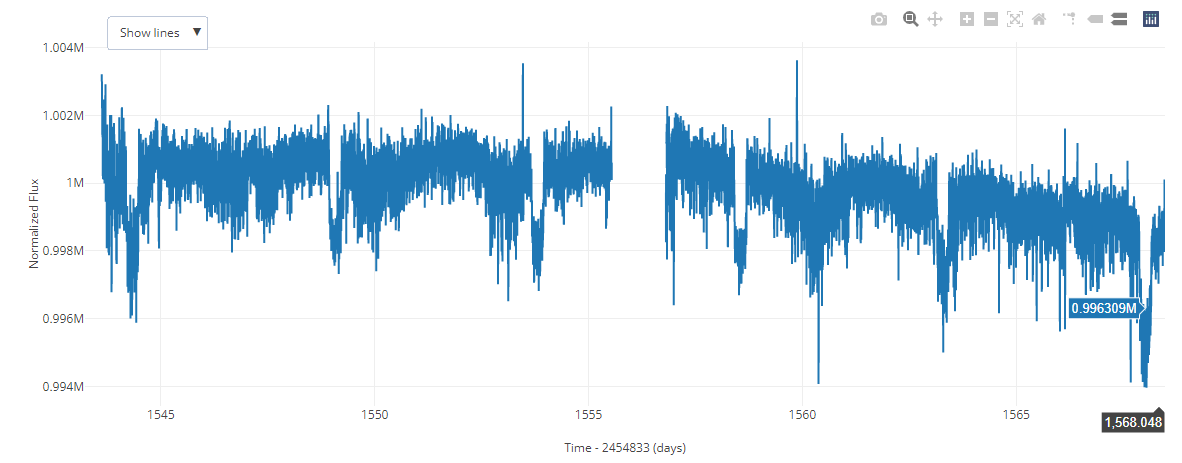Light Curve of KELT-11b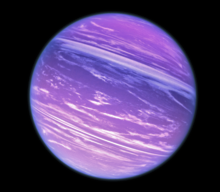KELT-11b virtual representation

### GJ 676 A e - Ara

Radius: Rp = √ 1 - 0,9886 · 0.69 = 0,0736718RJ · 100 = 7,36718% more big than the earth radius. Compared to academic google results and NASA files, the radius is 0,0736718RJ and the mass is 6.7MJ.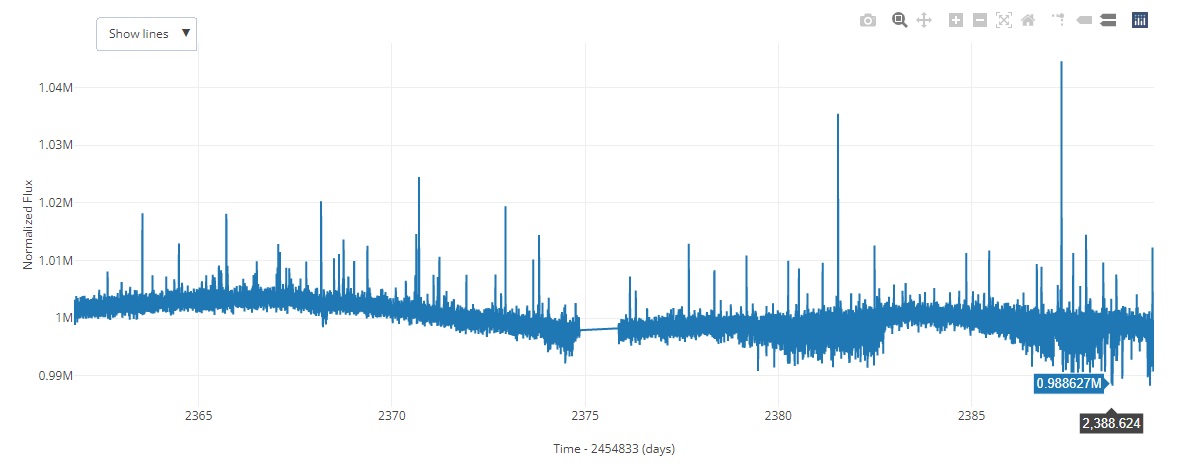Light Curve of GJ 676 A eGJ 676 A e virtual representation

### HD 43691 A b - Auriga

Radius: Rp = √ 1 - 0,8649 · 1.60 = 0,588095RJ · 100 = 58,8095% more big than the earth radius. Compared to academic google results and NASA files, the radius is 0,588095RJ and the mass is 1.38MJ.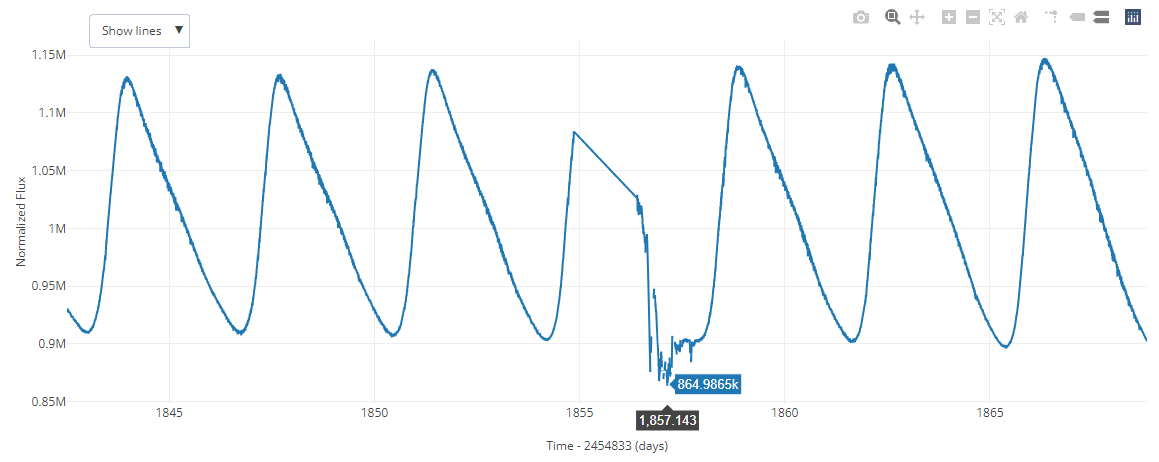Light Curve of HD 43691 A b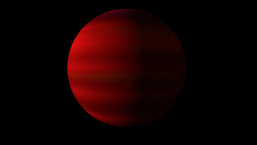HD 43691 A b virtual representation

## Creation of linear regression with the RStudio program

To finish with this section, we use the RStudio program to be able to generate a regression line. This will provide us with a series of coefficients and data that will delimit the mass of our planet.

First, you had to go to a NASA exoplanet finder, and select a total of 78 of these. Then, converting the file into csv, we upload it to RStudio, and write the following codes.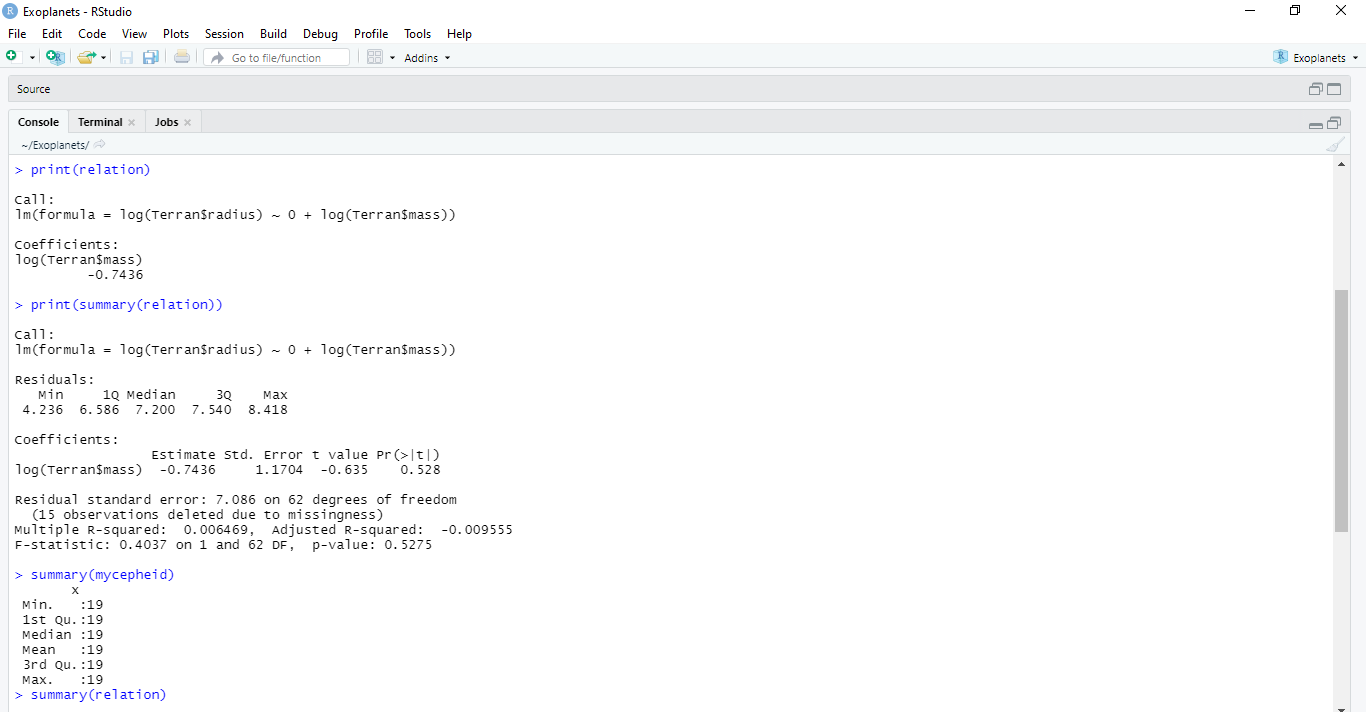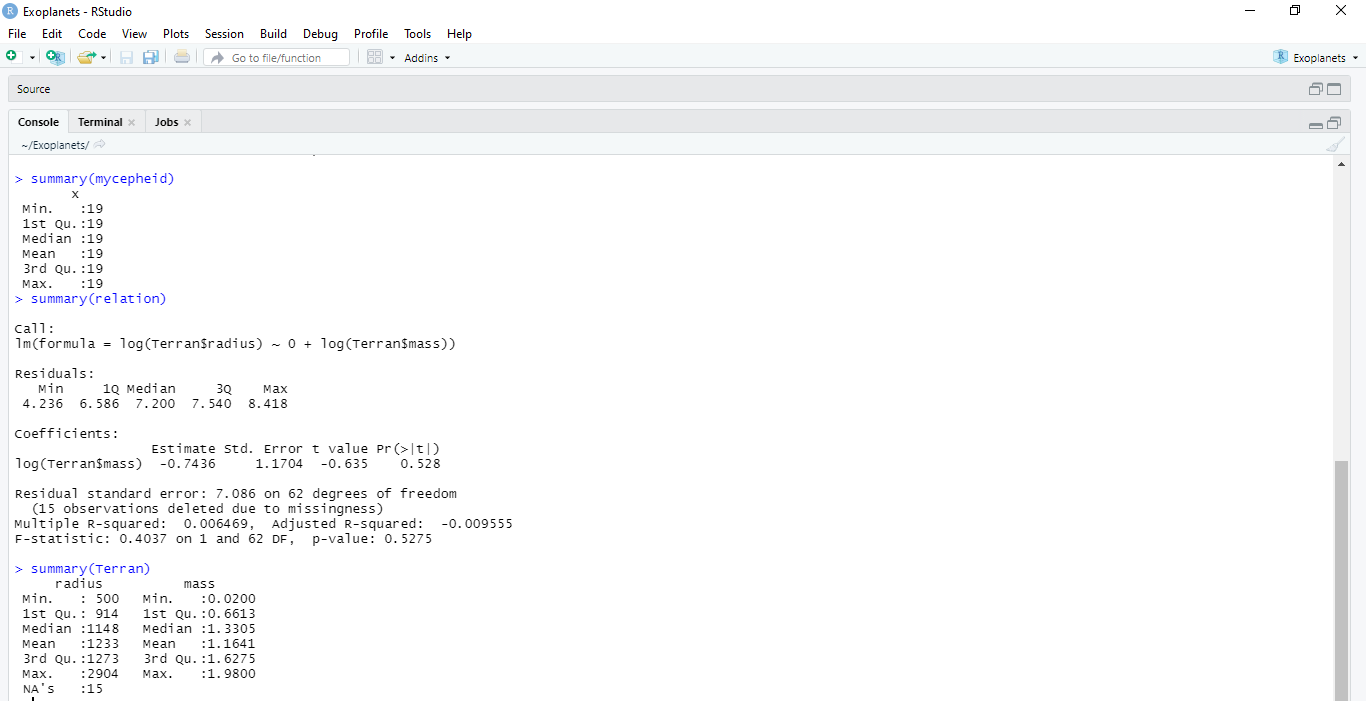With them a graph or diagram was obtained, which was not valid, so we opted to use the coefficients provided by the codes. Finally, with them you have to make a calculation of logarithms, which consists of: log (radius) = coefficient log (mass). If we substitute, it would be this: log (1148) = (-0,7436) log (x), what would it give 7,67295·10^-5, the mass of my exoplanet.